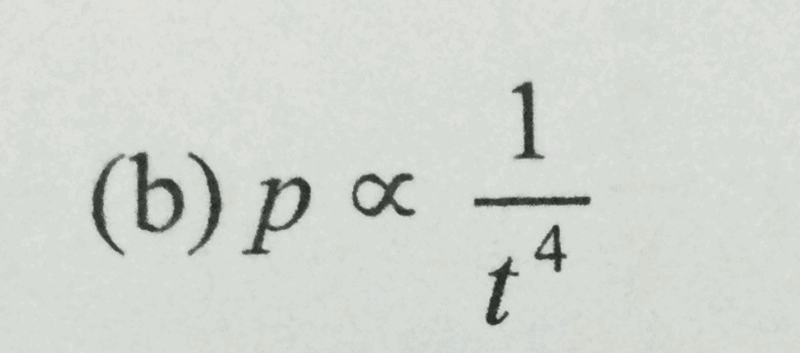# Question

hmmm okay,

p varies directly as q

Hence, p = kq  (k as a constant)

q = p/k —— (1)

√q varies inversely as t^2

Hence,  √q = k/t^2

you square the equation

q = k^2/t^4 ——- (2)

in this case let k =1, and sub equation (1) and (2) together, you will get

p=1/t^4

0 Replies 2 Likes ✔Accepted Answerthe answer is weirdly this aha🤠